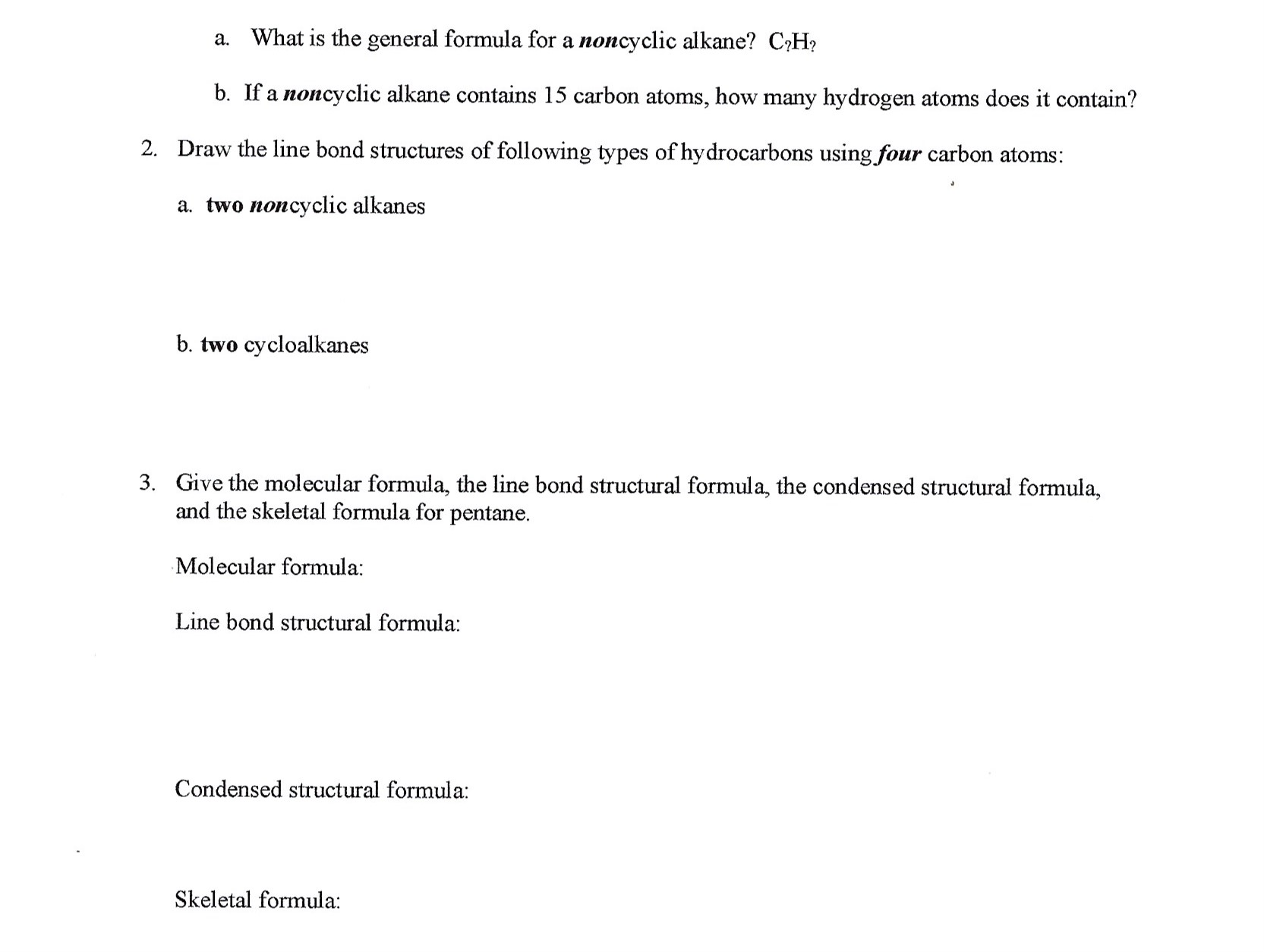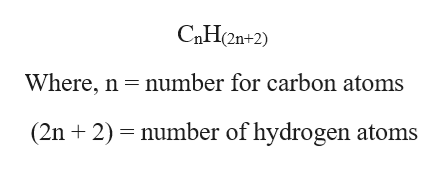# What is the general formula for a noncyclic alkane? C?Ha.b. If a noncyclic alkane contains 15 carbon atoms, how many hydrogen atoms does it contain?Draw the line bond structures of following types of hydrocarbons using four carbon atoms:2.a. two noncyclic alkanesb. two cycloalkanesGive the molecular formula, the line bond structural formula, the condensed structural formula,and the skeletal formula for pentane3.Molecular formula:Line bond structural formula:Condensed structural formula:Skeletal formula

Question
3 viewshelp_outlineImage TranscriptioncloseWhat is the general formula for a noncyclic alkane? C?H a. b. If a noncyclic alkane contains 15 carbon atoms, how many hydrogen atoms does it contain? Draw the line bond structures of following types of hydrocarbons using four carbon atoms: 2. a. two noncyclic alkanes b. two cycloalkanes Give the molecular formula, the line bond structural formula, the condensed structural formula, and the skeletal formula for pentane 3. Molecular formula: Line bond structural formula: Condensed structural formula: Skeletal formula fullscreen
check_circle

Step 1

As you have not mention which question is needed to be answered and since there are multiple questions, we are authorized to answer only one question, so we are providing the answer for the first question only. If you require the answer of the other questions, please post them separately again.

Step 2

(a) The general formula for a...help_outlineImage TranscriptioncloseCaH 2n+2) Where, n number for carbon atoms (2n + 2) number of hydrogen atoms fullscreen

### Want to see the full answer?

See Solution

#### Want to see this answer and more?

Solutions are written by subject experts who are available 24/7. Questions are typically answered within 1 hour.*

See Solution
*Response times may vary by subject and question.
Tagged in

### Organic Chemistry